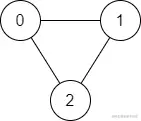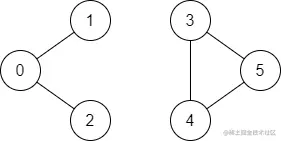# leetcode 1971. Find if Path Exists in Graph（python）

2022-01-31 09:33:17

「 This is my participation 11 The fourth of the yuegengwen challenge 11 God , Check out the activity details ：2021 One last more challenge

### describe

There is a bi-directional graph with n vertices, where each vertex is labeled from 0 to n - 1 (inclusive). The edges in the graph are represented as a 2D integer array edges, where each edges[i] = [ui, vi] denotes a bi-directional edge between vertex ui and vertex vi. Every vertex pair is connected by at most one edge, and no vertex has an edge to itself.

You want to determine if there is a valid path that exists from vertex start to vertex end.

Given edges and the integers n, start, and end, return true if there is a valid path from start to end, or false otherwise.

Example 1:``````Input: n = 3, edges = [[0,1],[1,2],[2,0]], start = 0, end = 2
Output: true
Explanation: There are two paths from vertex 0 to vertex 2:
- 0 → 1 → 2
- 0 → 2
Copy code ``````

Example 2:``````Input: n = 6, edges = [[0,1],[0,2],[3,5],[5,4],[4,3]], start = 0, end = 5
Output: false
Explanation: There is no path from vertex 0 to vertex 5.
Copy code ``````

Note:

• 1 <= n <= 2 * 10^5
• 0 <= edges.length <= 2 * 10^5
• edges[i].length == 2
• 0 <= ui, vi <= n - 1
• ui != vi
• 0 <= start, end <= n - 1
• There are no duplicate edges.
• There are no self edges.

### analysis

According to the meaning , Give an example with n A two-way graph of vertices , Each vertex is marked as 0 To n-1 A number in . The edges in the figure are represented as two-dimensional integer array edges , Each of them edges[i] = [ui, vi] It means a vertex ui And vertex vi Two way edges between . Each vertex pair is connected by at most one edge , And there are no edges with vertices connected to itself . The question asks to determine whether there is from the vertex start To the top end The effective path . Give edges and integers n、start and end , If there is a valid path from start to end , Then return to true, Otherwise return to false.

Use it directly BFS Ideas ：

• If start be equal to end Go straight back to True （ I didn't expect this border situation , It led to a wrong submission ！！）
• Will all edges There is a two-dimensional list of vertices in graph ,graph The length is n
• Initializes a length of n It's all about False Of visited list , Indicates whether a vertex has been accessed , If visited, it becomes True
• Initialize a queue stack , Store that has not been accessed and may be end The summit of , Add a vertex start
• When stack When it's not empty while loop , Take out stack The first element of is key , take visited[key] Set as True Indicates that you have been visited , If end stay graph[key] There has been a direct return in True , Otherwise it would be graph[key] Elements in that have not been accessed are added to stack in , Continue to cycle
• No legal path found at the end of the loop , Go straight back to False

``````class Solution(object):
def validPath(self, n, edges, start, end):
"""
:type n: int
:type edges: List[List[int]]
:type start: int
:type end: int
:rtype: bool
"""
if start == end: return True
graph = [[] for i in range(n)]
for s,e in edges:
graph[s].append(e)
graph[e].append(s)
visited = [False for _ in range(n)]
stack = [start]
while stack:
key = stack.pop(0)
visited[key] = True
if end in graph[key]:
return True
for vertex in graph[key]:
if not visited[vertex]:
stack.append(vertex)
return False

Copy code ``````

### Running results

``````Runtime: 1612 ms, faster than 97.22% of Python online submissions for Find if Path Exists in Graph.
Memory Usage: 110.9 MB, less than 89.03% of Python online submissions for Find if Path Exists in Graph.
Copy code ``````